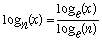home | O'Reilly's CD bookshelfs | FreeBSD | Linux | Cisco | Cisco Exam

### 2.13. Taking Logarithms

### Problem

You want to take a logarithm in various bases.

### Solution

For logarithms to base e , use the built-in ``` log``` :

`\$log_e = log(VALUE);`

For logarithms to base 10, use the POSIX module's ``` log10``` function:

```use POSIX qw(log10);
\$log_10 = log10(VALUE);```

For other bases, use the mathematical identity:where x is the number whose logarithm you want, n is the desired base, and e is the natural logarithm base.

```sub log_base {
my (\$base, \$value) = @_;
return log(\$value)/log(\$base);
}```

### Discussion

``` ``` The ``` log_base``` function lets you take logarithms to any base. If you know the base you'll want in advance, it's more efficient to cache the log of the base instead of recalculating it every time.

```# log_base defined as above
```

log10(10,000) = 4

```
```

The Math::Complex module does the caching for you via its ``` logn()``` routine, so you can write:

```use Math::Complex;
printf "log2(1024) = %lf\n", logn(1024, 2); # watch out for argument order!
```

log2(1024) = 10.000000

```
```

even though no complex number is involved here. This is not very efficient, but there are plans to rewrite ``` Math::Complex``` in C for speed.

The ``` log``` function in perlfunc (1) and Chapter 3 of Programming Perl ; the documentation for the standard POSIX module (also in Chapter 7 of Programming Perl )2.12. Calculating More Trigonometric Functions2.14. Multiplying Matrices